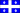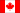# Unit conversion

### Imperial to and from Metric / decimal degree to sexagesimal degree

 Decimal Inch Millimetre Decimal Inch Centimetre Decimal Feet Metre Decimal Ounce Gram Decimal Pound Gram Decimal Pound Kilogram US Ton Kilogram US Ton Metric Ton UK Ton Kilogram UK Ton Metric Ton Farenheit Celcius Decimal Degree Sexagesimal Degree

### Glossary

Metic Weight Units:
1.000 000 000 t (one tonne)
1 000.000 000 kg (one thousand kilograms)
1 000 000.000 g (one million grams)
1 000 000 000 mg (one billion milligrams)
Imperial Weight Units:
1 US (short) Ton = 2000 lb = 32000 oz
1 UK (long) Ton = 2240 lb = 35840 oz
1 lb = 16 oz
1 oz = 0.0625 lb
Imperial and metric length:
1 foot = 12 inches = 304.8 millimeter = 30.48 centimeter
1 Inch = .0833 foot = 25.4 millimeter = 2.54 centimeter
1 meter = 100 centimeter = 1000 millimeter = 39.37 inch = (3' 33/8")
1 centimeter = 10 millimeter = .39370 inch = 0.0328 foot
1 millimeter = .1 centimeter = .03937 inch = 0.00328 foot
Abbreviations:
Inch; in
Foot; feet, ft
US Ton; short ton, sh tn, t
UK Ton; long ton, long tn, t
Ounce; oz
Pound; lb
Centimeter; cm
Millimeter; mm
Metric Ton; tonne, t, mt, M/T
Milligram; mg
kilogram; kg
Gram; g
Meter; m
Sexagesimal angle entry field (format example)
Example 1: 64°45'34" enter as 64d45m34s
Example 2: -30°5' enter as -30d5m
Example 3: 15°0'10" enter as 15d10s
Example 4: -0°0'50" enter as -50s

Last Updated: 15 February 2015

 Privacy policy |  Disclaimer |  TheoreticalMachinist.com Copyright © 2011-2018 Joly Concept